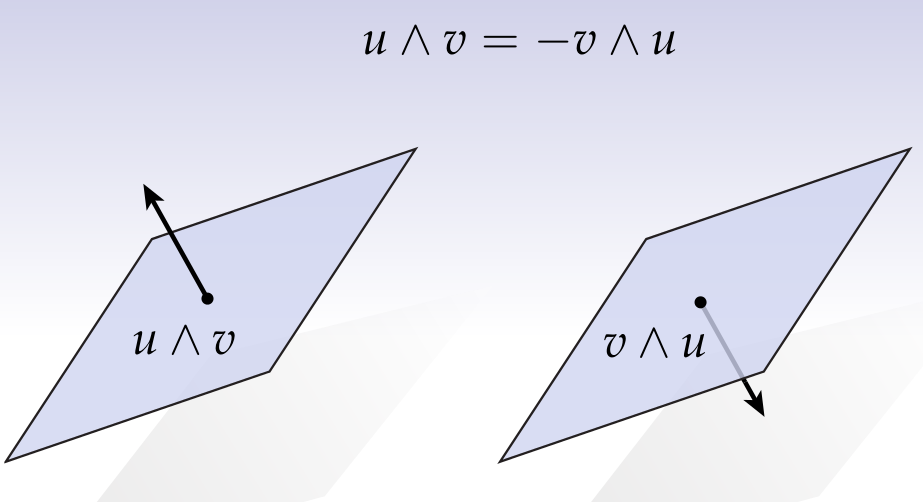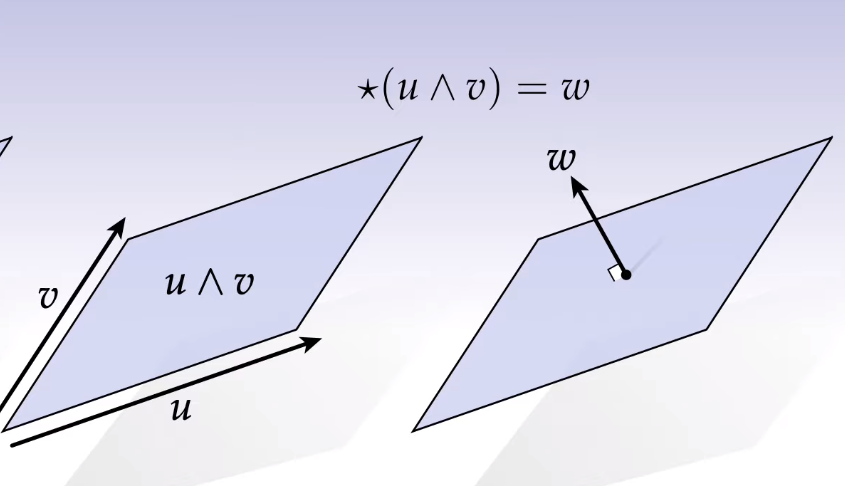# DDG Week 3

## Exterior Calculus

Why?

• Talk about signed volume
• Geometry -> Algebraic Geometry
• Geometric algebra (Clifford algebra, spin physics)
• Physics
• massless quantitiies are vectors
• massive quantities are forms

### discrete exterior calculus (DEC)

Work from linear algebra and vector calculus to Exterior Algebra and Exterior Calculus.

DEC -> Discretize all the above.

motivation: do cool stuff like solve PDEs on meshes.

Concepts:

• Poisson
• Helmholtz-Hodge
• homology
• cohomology

Applications:

• smoothing
• distance
• vector field
• parameterization
• meshing

### Wedge Product

span: the subspace that is a linear combination of the vectors.

Defn: In a vector space $V$, the span of a finite set of vectors $\{v_1,\dots,v_k\}$ is the set of all linear combinations:

$\text{span}(\{v_1,\dots,v_k\}) := \{x \in V | x = \sum_{i=1}^{k} a_i v_i, ~ a_i \in \mathbb{R}\}$

Wedge product:Oriented finite extent. Anti-symmetric.

note: $u \wedge u = 0$

$u \wedge v \wedge w = (u \wedge v) \wedge w = u \wedge (v \wedge w) \\ u \wedge (v_1 + v_2) = u \wedge v_1 + u \wedge v_2 \\$

The resulting object is a k-vector.

• 0-vector ?
• 1-vector $u$
• 2-vector $u \wedge v$ -> parallelogram (direction + magnitude)
• 3-vector $u \wedge v \wedge w$ -> volume + direction?

A k-vector with the same area and orientation is equivalent. Any "patch" with the same area and normal is equivalent.

For convenience, say a 0-vector is a scalar.

### Hodge Star

Orthogonal Compliment:

$V := \text{span}(\{u,v\}) \\ V^{\perp} := \{x \in \mathbb{R}^n | = 0 ~ \forall w \in V\}$

Defn Let $U \subset V$ be a linear subspace of a vector space $V$ with an inner product $<\cdot, \cdot>$. The orthogonal compliment of $U$ is the collection of vectors.

$U^{\perp} := \{v \in V | = 0 ~ \forall u \in U\}$

With a compliment, we can say things like "X except Y".

$\star (u \wedge v) = w$convention: $z \wedge \star z$ is positively oriented.

The 2-form is the "oriented planar segment" whereas the hodge star of a 2-vector gives us the normal to the surface (in $\mathbb{R}^3$).

### Coordinate Representation

Like the basis of linear algebra, we can find the basis of $V$ by thinking of the wedges:

e.g. in $\mathbb{R}^4 \quad \mathcal{B} = \{e_1,e_2,e_3,e_4\}$

$e_1 \wedge e_2 \\ e_1 \wedge e_3 \quad e_2 \wedge e_3 \\ e_1 \wedge e_4 \quad e_2 \wedge e_4 \quad e_3 \wedge e_4$

The wedges $e_2 \wedge e_1 = e_1 \wedge e_2$ are omitted, so in general only $e_i \wedge e_j \quad i < j$ are considered. Also, with 3-vectors we have:

$e_1 \wedge e_2 \wedge e_3 \\ e_1 \wedge e_2 \wedge e_3 \\ e_1 \wedge e_3 \wedge e_4 \\ e_2 \wedge e_3 \wedge e_4$

so the same general rule applies where we take all permutations such that $i < j < k$. We get $\text{dim}(V) ~ \text{choose} ~ k$ basis k-vectors for a vector space $V$.